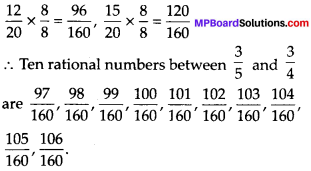## MP Board Class 8th Maths Solutions Chapter 1 Rational Numbers Ex 1.2

Question 1.
Represent these numbers on the number line.
(i) $$\frac{7}{4}$$
(ii) $$\frac{-5}{6}$$
Solution:
(i) We have to represent $$\frac{7}{4}$$ on the number line. $$\frac{7}{4}$$ can be written as $$1 \frac{3}{4} \cdot 1 \frac{3}{4}$$ lies between 1 and 2.
Step-1: Draw a number line and mark O on it to represents ‘0’ (zero)
Step-2 : Take a point A to represent
1 and B to represent 2.
Step-3 : Divide the distance of A and B in four equal parts A A1, A1A2, A2A3, A3B.
Step-4 : Count from 1 and reach to the third point A3 and A3 is the required point on number line.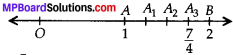(ii) $$\frac{-5}{6}$$ is lies between 0 and -1.
Step-1: Draw a number line and mark O on it to represent ‘0’ (zero).
Step-2 : Take a point A to represent -1.
Step-3 : Divide the distance of A and O in six equal parts AO5, O5O4, O4O3, O3O2, O2O1, O1O.
Step-4 : Count from 0 and reach to the fifth point O5.
O5 is the required point.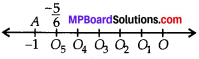Question 2.
Represent $$\frac{-2}{11}, \frac{-5}{11}, \frac{-9}{11}$$ on the number line.
Solution:
We have to mark $$\frac{-2}{11}, \frac{-5}{11}, \frac{-9}{11}$$ on the same number line.
Since $$\frac{-2}{11}, \frac{-5}{11}, \frac{-9}{11}$$ all are less than 0 but greater than -1.
∴ All these lie between 0 and -1.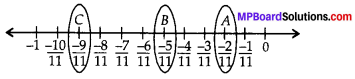Thus, A, B and C are the required points.

Question 3.
Write five rational numbers which are smaller than 2.
Solution:
Five numbers less than 2 lies on the left of 2 on the number line.
∴ Five rational numbers are $$0, \frac{1}{5}, \frac{2}{5}, \frac{3}{5}, \frac{4}{5}$$Question 4.
Find ten rational numbers between $$\frac{-2}{5}$$ and $$\frac{1}{2}$$.
Solution:
We have given, two rational numbers $$\frac{-2}{5}$$ and $$\frac{1}{2}$$.
First we make the same denominator of both rational numbers.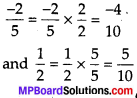Now, we have to find 10 rational numbers between $$\frac{-4}{10}$$ and $$\frac{5}{10}$$. so we have to multiply the numerator and denominator by a number such that difference between numerators is atleast 10.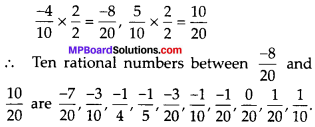Question 5.
Find five rational numbers between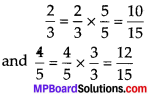Solution:
First we make the same denominator of both rational numbers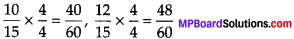Since, we have to find five rational numbers between $$\frac{10}{15}$$ and $$\frac{12}{15}$$ so we multiply the numerator and denominator by a number such that difference between the numerators is atleast 5.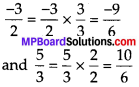∴ The five rational numbers between $$\frac{1}{4}$$ and $$\frac{2}{4}$$ are $$\frac{41}{60}, \frac{42}{60}, \frac{43}{60}, \frac{44}{60}, \frac{45}{60}$$

(ii) First we make the same denominator of both rational numbers.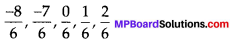Since, we have to find five rational numbers between and $$-\frac{9}{6}$$ and $$\frac{10}{6}$$, so we do not need to multiply the numerator and denominator of $$-\frac{9}{6}$$ and $$\frac{10}{6}$$ by any number, because we can see that the difference between the numerators is 19 > 5.
∴ Five rational numbers between $$\frac{-3}{2}$$ and $$\frac{5}{3}$$ are $$\frac{-8}{6}, \frac{-7}{6}, \frac{0}{6}, \frac{1}{6}, \frac{2}{6}$$

(iii) First we make the same denominator of both rational numbers.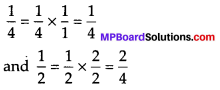Since we have to find five rational numbers between $$\frac{1}{4}$$ and $$\frac{2}{4}$$, so we multiply the numerator and denominator by a number such that difference between the numerators is atleast 5.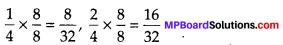∴ Five rational numbers between $$\frac{1}{4}$$ and $$\frac{2}{4}$$ are $$\frac{9}{32}, \frac{10}{32}, \frac{11}{32}, \frac{12}{32}, \frac{13}{32}$$.

Question 6.
Write five rational numbers greater than -2.
Solution:
Five rational numbers greater than -2 lies on the right side of -2 on number line.
∴ Any five rationals on the right of -2 are $$\frac{-3}{2},-1, \frac{-1}{2}, 0, \frac{1}{2}$$.Question 7.
Find ten rational numbers between $$\frac{3}{5}$$ and $$\frac{3}{4}$$
Solution:
Make the common denominator.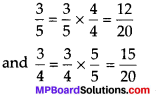Since we have to find ten rational numbers between $$\frac{3}{5}$$ and $$\frac{3}{4}$$ so, we multiply the numerator and denominator by a number such that difference between the numerators is atleast 10.